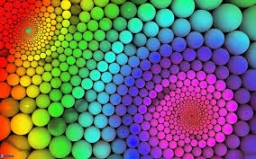How many times does the surface of a sphere decrease if we reduce its radius twice?

n =  4

### Step-by-step explanation:Did you find an error or inaccuracy? Feel free to write us. Thank you!Tips to related online calculators
Need help to calculate sum, simplify or multiply fractions? Try our fraction calculator.
Check out our ratio calculator.

#### You need to know the following knowledge to solve this word math problem:

We encourage you to watch this tutorial video on this math problem:

## Related math problems and questions:

• Volume of sphereHow many times does the volume of a sphere increase if its radius increases 2 ×?
• Surface of wallFind by what percentage the surface of the cube will decrease if we reduce the surface of each of its walls by 12%.The radius of the sphere we reduce by 1/3 of the original radius. How much percent does the volume and surface of the sphere change?
• Cylinder twiceIf the radius of the cylinder increases twice, and the height is reduced twice, then the volume of the cylinder increases (how many times?):
• Ratio of volumesIf the heights of two cylindrical drums are in the ratio 7:8 and their base radii are in the ratio 4:3. What is the ratio of their volumes?The surface of the sphere is 60 cm square. Calculate its radius; result round to tenth of cm.
• The EarthThe Earth's surface is 510,000,000 km2. Calculates the radius, equator length, and volume of the Earth, assuming the Earth has the shape of a sphere.
• Area of rectangleHow many times will increase the area of the rectangle if we increase twice the length and at the same time we decrease the width by half?
• Area to volumeIf the surface area of a cube is 486, find its volume.
• Cube wallsFind the volume and the surface area of the cube if the area of one of its walls is 40 cm2.
• Sphere VSFind the surface and volume of a sphere that has a radius of 2 dm.
• Cone and the ratioThe rotational cone has a height 43 cm, and the ratio of the base surface to lateral surface is 5: 7. Calculate the surface of the base and the lateral surface.Determine the radius of the circle if its perimeter and area is the same number.Calculate the volume of the cube if its surface is 150 cm2.The cuboid has dimensions in ratio 4: 3: 5, the shortest edge is 12 cm long. Find: (A) the lengths of the remaining edges, (B) the surface of the cuboid, (C) the volume of the cuboidThe volume of the sphere is 1 m3, what is its surface?How many times will increase if the surface area of the cube if we triple length of its edge?